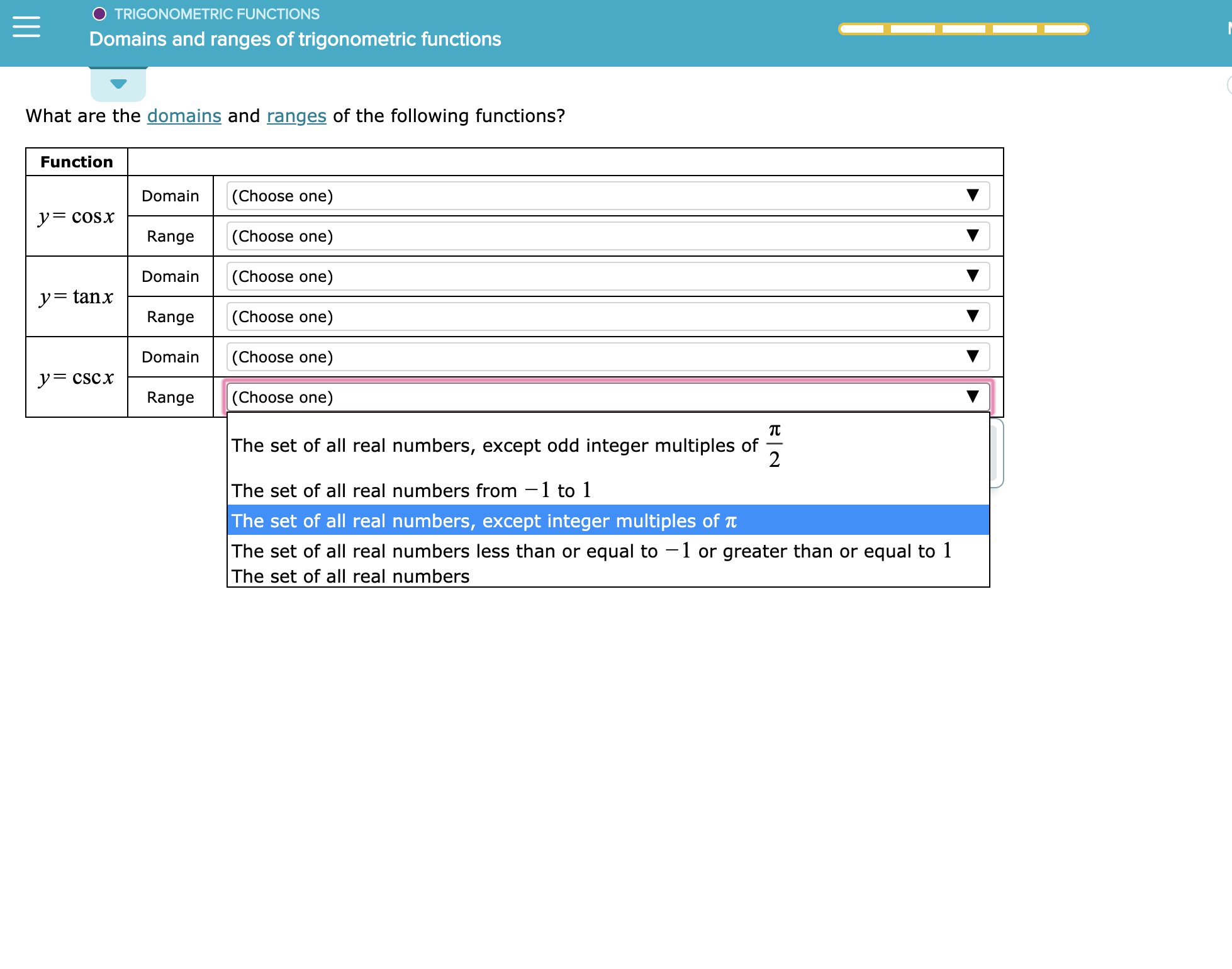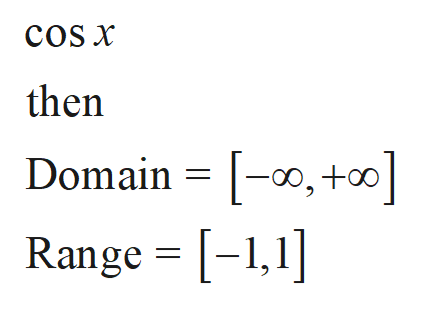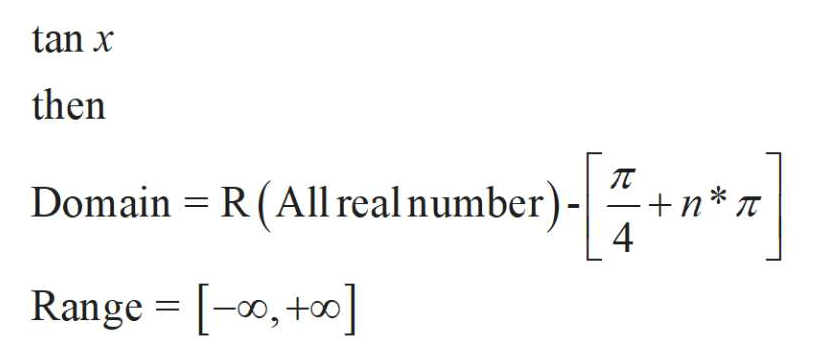TRIGONOMETRIC FUNCTIONSDomains and ranges of trigonometric functionsWhat are the domains and ranges of the following functions?Function(Choose one)DomainycoSx(Choose one)RangeDomain(Choose one)y=tanx(Choose one)RangeDomain(Choose one)yCSCx(Choose one)RangeTCThe set of all real numbers, except odd integer multiples of2The set of all real numbers from 1 to 1The set of all real numbers, except integer multiples of TThe set of all real numbers less than or equal to 1 or greater than or equal to 1The set of all real numbers

Question

See attachmenthelp_outlineImage TranscriptioncloseTRIGONOMETRIC FUNCTIONS Domains and ranges of trigonometric functions What are the domains and ranges of the following functions? Function (Choose one) Domain ycoSx (Choose one) Range Domain (Choose one) y=tanx (Choose one) Range Domain (Choose one) yCSCx (Choose one) Range TC The set of all real numbers, except odd integer multiples of 2 The set of all real numbers from 1 to 1 The set of all real numbers, except integer multiples of T The set of all real numbers less than or equal to 1 or greater than or equal to 1 The set of all real numbers fullscreen
Step 1

Consider the given functions

Step 2

The consider the first functionhelp_outlineImage TranscriptionclosecoS x then Domain =0, + Range [-1,] fullscreen
Step 3

Consider the Secon...help_outlineImage Transcriptionclosetan x then Domain R (All real number) -|+n*T 4 Range [, fullscreen

Want to see the full answer?

See Solution

Want to see this answer and more?

Our solutions are written by experts, many with advanced degrees, and available 24/7

See Solution
Tagged in

Other